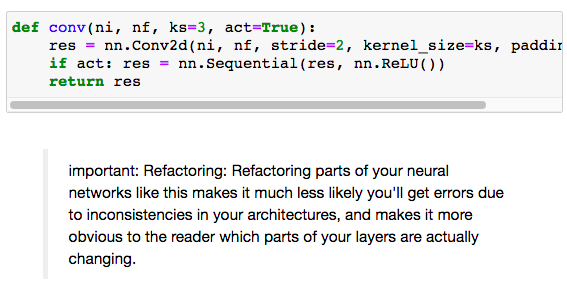# Lesson 13 def conv

There was a def made in Lesson 13 for which I need some help understanding:Questions:

1. Will the format of this network be
Sequential(
nn.Conv2d(),
nn.ReLU(),
nn.Conv2d(),
nn.ReLU()
?

2. What is act? When is it False?

3. At what point will the res be returned? Until the size is 1 x 1?

4. If the code was not refactored, how will it be written?

5. Based on the note: which layer is changing?

Maria

if def() was called with act=True
`Sequential( nn.Conv2d(), nn.ReLU() `
if def() was called with act=False
`Sequential( nn.Conv2d() `

It’s an argument you pass in when you call the method def()
If you pass in a True, this method will add an “activation function” at the end. ReLU is the activation function it adds.
like in the book few lines down

``````simple_cnn = sequential(
conv(1 ,4),            #14x14
conv(4 ,8),            #7x7
conv(8 ,16),           #4x4
conv(16,32),           #2x2
conv(32,2, act=False), #1x1
Flatten(),
)
``````

This is a method/function so, res is returned at the end of the function.
This method just aids is building the model not actually running it.

@meanpenguin Thank you – I understand it better now!

Maria